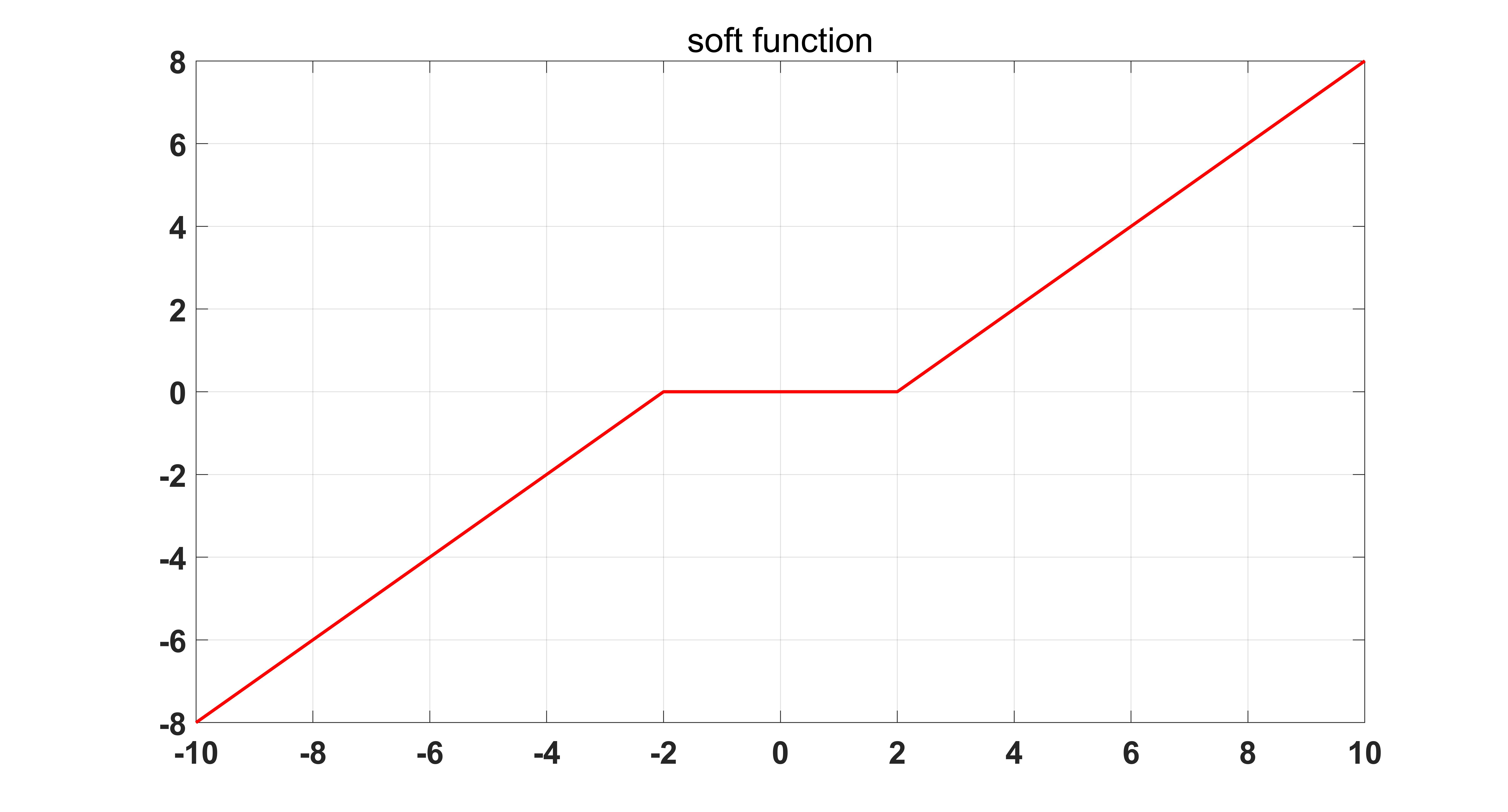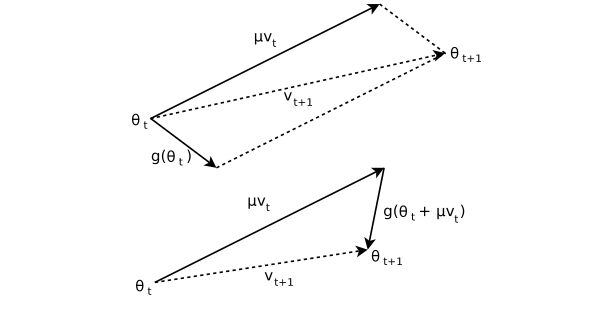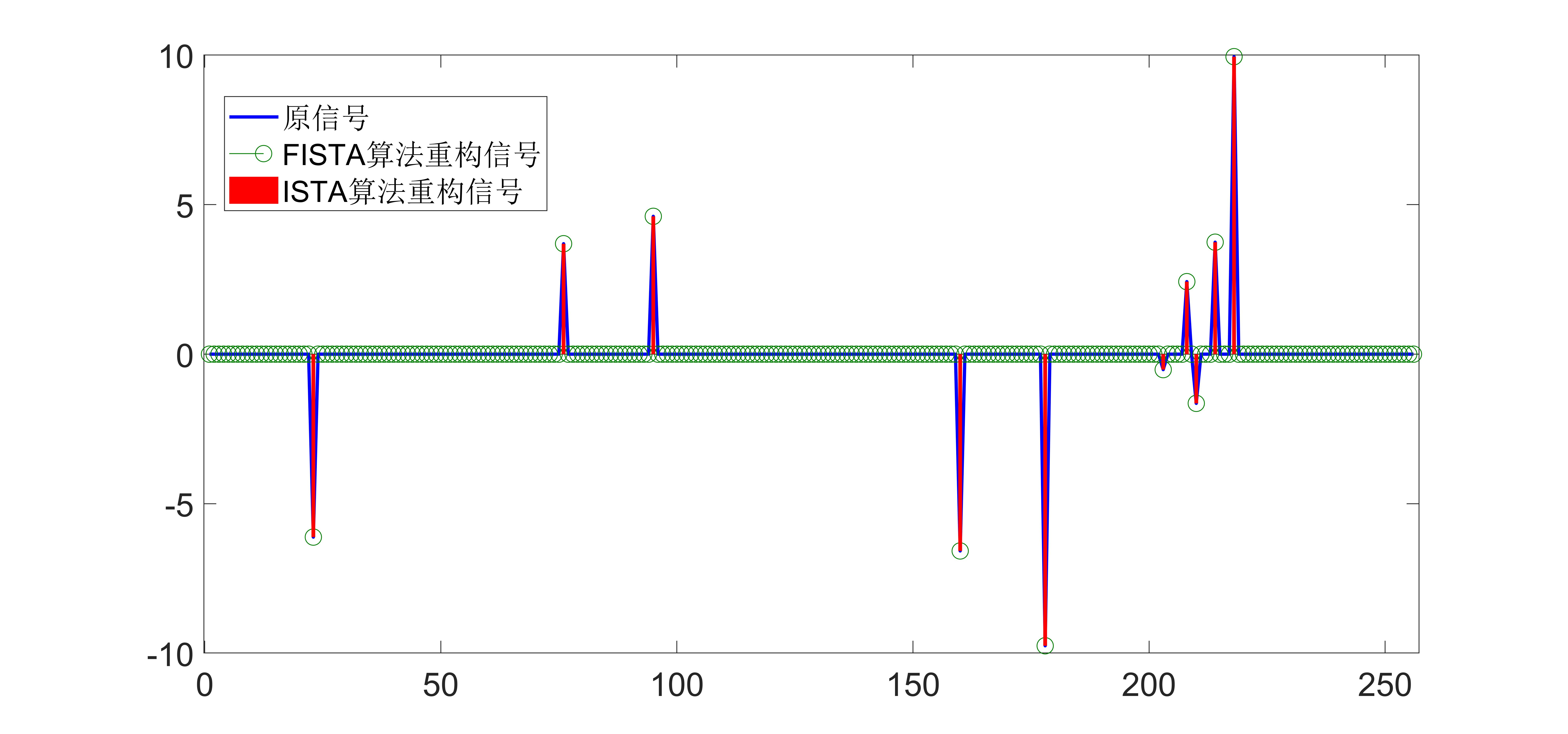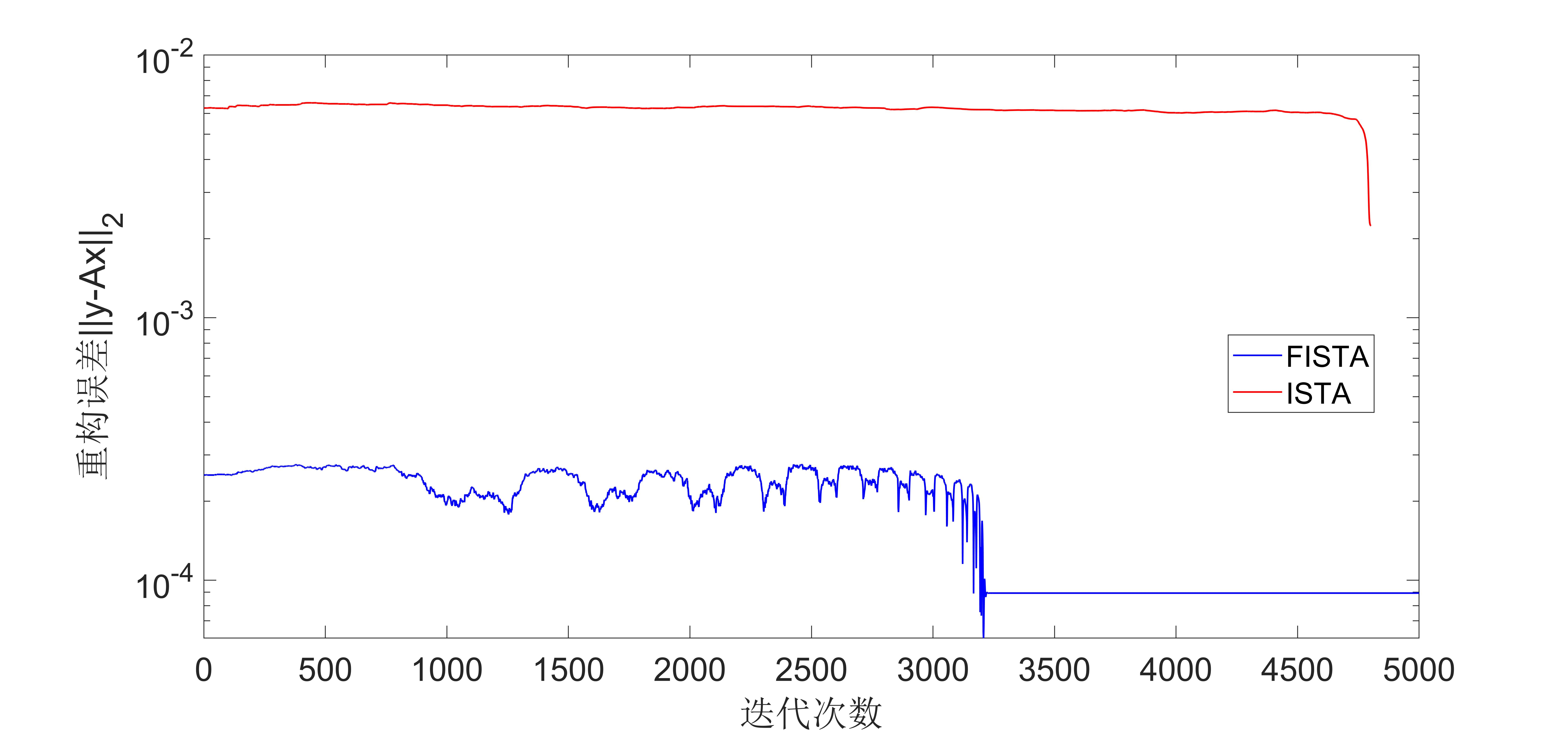• FISTA算法是对ISTA算法的优化提升，本程序是在ISTA算法的基础上进行的编程改进。
• 去噪算法基于小波变换的FISTA算法基于小波变换的FISTA算法
• 利用fista算法进行图像复原的matlab源码。
• FISTA算法的代码运行！ FISTA算法的代码运行！ 自己有fista算法代码，有没有大佬知道fista算法代码怎么跑，下载的代码有点问题自己也不知道怎么改，求大佬带带啊，可以一起加个好友一起讨论，欢迎私信，欢迎一起交流...
FISTA算法的代码运行！
FISTA算法的代码运行！ 自己有fista算法代码，有没有大佬知道fista算法代码怎么跑，下载的代码有点问题自己也不知道怎么改，求大佬带带啊，可以一起加个好友一起讨论，欢迎私信，欢迎一起交流，本人小白一枚！
展开全文深度学习
• 此函数用FISTA算法解决压缩感知，注释很详细，里面包含测试图片MATLAB
• ISTA 和 FISTA优化算法 据https://zhuanlan.zhihu.com/p/31232568?from_voters_page=true
ISTA 和 FISTA优化算法
据https://zhuanlan.zhihu.com/p/31232568?from_voters_page=true
展开全文• ## 终于搞定了FISTA算法

千次阅读 热门讨论 2012-02-16 15:23:54
matlab的代码，查了半天，发现原来是循环中少更新了x_k变量，内牛满面啊。
matlab的代码，查了半天，发现原来是循环中少更新了x_k变量，内牛满面啊。

展开全文matlab
• ISTA算法和FISTA算法是求解线性逆问题的经典方法，隶属于梯度类算法，也常用于压缩感知重构算法中，隶属于梯度类算法，这次将这2中算法原理做简单分析，并给出matlab仿真实验，通过实验结果来验证算法性能。...

缺月挂疏桐，漏断人初静。 谁见幽人独往来，缥缈孤鸿影。 惊起却回头，有恨无人省。 拣尽寒枝不肯栖，寂寞沙洲冷。---- 苏轼
更多精彩内容请关注微信公众号 “优化与算法”

ISTA算法和FISTA算法是求解线性逆问题的经典方法，隶属于梯度类算法，也常用于压缩感知重构算法中，隶属于梯度类算法，这次将这2中算法原理做简单分析，并给出matlab仿真实验，通过实验结果来验证算法性能。

1. 引言
对于一个基本的线性逆问题:

y

=

A

x

+

w

(

1

)

其中

A

∈

M

×

N

{\bf{A }} \in {^{M \times N}}

,

y

∈

M

{\bf{y }} \in {^{M}}

且是已知的，

w

\bf{w}

是未知噪声。 (1)式可用最小二乘法（Least Squares）来求解:

x

^

L

S

=

arg

⁡

m

i

x

n

∥

A

x

−

y

∥

2

2

(

2

)

当

M

=

N

M=N

且

A

\bf{A}

非奇异时，最小二乘法的解等价于

A

−

1

y

\bf{A^{-1}y}

。
然而，在很多情况下，

(

A

)

\bf(A)

是病态的（ill-conditioned），此时，用最小二乘法求解时，系统微小的扰动都会导致结果差别很大，可谓失之毫厘谬以千里，因此最小二乘法不适用于求解病态方程。

**什么是条件数？**矩阵

A

\bf{A}

的条件数是指

A

\bf{A}

的最大奇异值与最小奇异值的比值，显然条件数最小为1，条件数越小说明矩阵越趋于“良态”，条件数越大，矩阵越趋于奇异，从而趋于“病态”。

x

^

T

=

arg

⁡

m

i

x

n

∥

A

x

−

y

∥

2

2

+

λ

∥

x

∥

2

2

(

3

)

其中

λ

>

0

\lambda>0

为正则化参数。问题(3)的解等价于如下岭回归估计器：

x

^

T

=

(

x

T

x

+

λ

I

)

−

1

x

T

y

(

4

)安德烈·尼古拉耶维奇·蒂霍诺夫（俄文：阿尔瓦勒德普列耶娃；1906年10月17日至1993年10月7日）是苏联和俄罗斯数学家和地球物理学家，以对拓扑学、泛函分析、数学物理和不适定问题的重要贡献而闻名。他也是地球物理学中大地电磁法的发明者之一。

岭回归是采用

ℓ

2

{\ell _2}

范数作为正则项，另一种求解式(1)的方法是采用

ℓ

1

{\ell _1}

范数作为正则项，这就是经典的LASSO(Least absolute shrinkage and selection operator)问题：

x

^

=

arg

⁡

m

i

x

n

∥

A

x

−

y

∥

2

2

+

λ

∥

x

∥

1

(

5

)

{\bf{\hat x}} = \mathop {\arg {\mathop{\rm mi}\nolimits} }\limits_{\bf{x}} n\left\| {{\bf{Ax}} - {\bf{y}}} \right\|_2^2 + \lambda {\left\| {\bf{x}} \right\|_1}~~~~~~~~~~~~~~~~~~~~~~~~~(5)

采用

ℓ

1

{\ell _1}

范数正则项相对于

ℓ

2

{\ell _2}

范数正则项有两个优势，第一个优势是

ℓ

1

{\ell _1}

范数正则项能产生稀疏解，第二个优势是其具有对异常值不敏感的特性，这一点恰好与岭回归相反。
式(5)中的问题是一个凸优化问题，通常可以转化为二阶锥规划（second order cone programming）问题，从而使用内点法（interior point）等方法求解。然而在大规模问题中，由于数据维度太大，而内点法的算法复杂度为

O

(

N

3

)

O({N^3})

，导致求解非常耗时。
基于上述原因，很多研究者研究通过简单的基于梯度的方法来求解（5）式。基于梯度的方法其计算量主要集中在矩阵

A

\bf{A}

与向量

y

\bf{y}

的乘积上，算法复杂度小，而且算法结构简单，容易操作。
2. 迭代收缩阈值算法（ISTA）
在众多基于梯度的算法中，迭代收缩阈值算法（Iterative Shrinkage Thresholding Algorithm）是一种非常受关注的算法，ISTA算法在每一次迭代中通过一个收缩/软阈值操作来更新

x

\bf{x}

，其具体迭代格式如下：

x

k

+

1

=

s

o

f

t

λ

t

(

x

k

−

2

t

A

T

(

A

x

k

−

y

)

)

(

6

)

{{{\bf{x}}_{k + 1}} = {{\mathop{\rm soft}\nolimits} _{\lambda t}}({{\bf{x}}_k} - 2t{{\bf{A}}^{\rm T}}({\bf{A}}{{\bf{x}}_k} - {\bf{y}}))}~~~~~~~~~~~~~~~~~~(6)

其中

s

o

f

t

λ

t

(

⋅

)

{{\mathop{\rm soft}\nolimits} _{\lambda t}}( \cdot )

是软阈值操作函数：

s

o

f

t

T

(

x

)

=

s

i

g

n

(

x

i

)

(

∣

x

i

∣

−

T

)

(

7

)

{{\mathop{\rm soft}\nolimits} _T}({{\bf{x}}_{\rm{}}}) = sign({x_i})(\left| {{x_i}} \right| - T)~~~~~~~~~~~~~~~~~~~~~~~~~~~(7)

软阈值操作函数如下图所示：其中

s

i

g

n

(

)

sign()

是符号函数。
考虑一个连续可导的无约束最小化问题：

min

⁡

{

f

(

x

)

:

x

∈

R

N

}

(

8

)

\min \{ f({\bf{x}}):{\bf{x}} \in {R^N}\} ~~~~~~~~~~~~~~~~~~~~~~~~~~~~~~~~~~~(8)

(8)式可用梯度下降法来求解：

x

0

∈

R

N

,

x

k

=

x

k

−

1

−

t

k

∇

f

(

x

k

−

1

)

(

9

)

{{\bf{x}}_0} \in {R^N},~~~~~{{\bf{x}}_k} = {{\bf{x}}_{k - 1}} - {t_k}\nabla f({{\bf{x}}_{k - 1}})~~~~~~~~~~~~~~~~~(9)

这里

t

k

>

0

t_k>0

是迭代步长。我们知道，梯度下降法可以表示成

f

f

在点

x

k

−

1

x_{k-1}

处的近端正则化（proximal regularization），其等价形式可以表示为：

x

k

=

arg

⁡

m

i

x

n

{

f

(

x

k

−

1

)

+

⟨

x

−

x

k

−

1

,

∇

f

(

x

k

−

1

)

⟩

+

1

2

t

k

∥

x

−

x

k

−

1

∥

2

2

}

(

10

)

{{\bf{x}}_k} = \mathop {\arg {\mathop{\rm mi}\nolimits} }\limits_{\bf{x}} n\left\{ {f({{\bf{x}}_{k - 1}}) + \left\langle {{\bf{x}} - {{\bf{x}}_{k - 1}},\nabla f({{\bf{x}}_{k - 1}})} \right\rangle + {1 \over {2{t_k}}}\left\| {{\bf{x}} - {{\bf{x}}_{k - 1}}} \right\|_2^2} \right\}~~~(10)

(9)-(10)可由李普希兹连续条件

∥

∇

f

(

x

k

)

−

∇

f

(

x

k

−

1

)

∥

2

≤

L

(

f

)

∥

x

k

−

x

k

−

1

∥

2

{\left\| {\nabla f({{\bf{x}}_k}) - \nabla f({{\bf{x}}_{k - 1}})} \right\|_2} \le L(f){\left\| {{{\bf{x}}_k} - {{\bf{x}}_{k - 1}}} \right\|_2}

和

f

f

在

x

k

−

1

x_{k-1}

处的2阶泰勒展开得到，很简单，这里不再赘述。

将(8)式加上

ℓ

1

{\ell _1}

范数正则项，得到：

min

⁡

{

f

(

x

)

+

λ

∥

x

∥

1

:

x

∈

R

N

}

(

11

)

\min \{ f({\bf{x}}) + \lambda {\left\| {\bf{x}} \right\|_1}:{\bf{x}} \in {R^N}\} ~~~~~~~~~~~~~~~~~~~(11)

则(10)式相应变成：

x

k

=

arg

⁡

m

i

x

n

{

f

(

x

k

−

1

)

+

⟨

x

−

x

k

−

1

,

∇

f

(

x

k

−

1

)

⟩

+

1

2

t

k

∥

x

−

x

k

−

1

∥

2

2

+

λ

∥

x

∥

1

}

(

12

)

{{\bf{x}}_k} = \mathop {\arg {\mathop{\rm mi}\nolimits} }\limits_{\bf{x}} n\left\{ {f({{\bf{x}}_{k - 1}}) + \left\langle {{\bf{x}} - {{\bf{x}}_{k - 1}},\nabla f({{\bf{x}}_{k - 1}})} \right\rangle + {1 \over {2{t_k}}}\left\| {{\bf{x}} - {{\bf{x}}_{k - 1}}} \right\|_2^2 + \lambda {{\left\| {\bf{x}} \right\|}_1}} \right\}~~(12)

时(12)忽略掉常数项

f

(

x

k

−

1

)

f(\bf x_{k-1})

和

∇

f

(

x

k

−

1

)

{\nabla f({{\bf{x}}_{k - 1}})}

之后，(12)式可以写成：

x

k

=

s

o

f

t

λ

t

k

(

x

k

−

1

−

t

k

∇

f

(

x

k

−

1

)

)

(

13

)

{{\bf{x}}_k} = {{\mathop{\rm soft}\nolimits} _{\lambda {t_k}}}({{\bf{x}}_{k - 1}} - {t_k}\nabla f({{\bf{x}}_{k - 1}})) ~~~~~~~~~~~~~~~~~~~~(13)

文献中已经证明，当迭代步长取

f

f

的李普希兹常数的倒数（即

1

L

(

f

)

{1 \over {L(f)}}

）时，由ISTA算法生成的序列

x

k

\bf x_k

的收敛速度为

O

(

1

k

)

O({1 \over {\rm{k}}})

，显然为次线性收敛速度。
ISTA算法的伪代码见原文，matlab代码如下：
function [x_hat,error] = cs_ista(y,A,lambda,epsilon,itermax)
% Iterative Soft Thresholding Algorithm(ISTA)
% Version: 1.0 written by Louis Zhang @2019-12-7
% Reference: Beck, Amir, and Marc Teboulle. "A fast iterative
% shrinkage-thresholding algorithm for linear inverse problems."
% SIAM journal on imaging sciences 2.1 (2009): 183-202.

% Inputs:
% y         - measurement vector
% A         - measurement matrix
% lambda    - denoiser parameter in the noisy case
% epsilon   - error threshold
% inter_max - maximum number of amp iterations
%
% Outputs:
% x_hat     - the last estimate
% error     - reconstruction error

if nargin < 5
itermax = 10000 ;
end
if nargin < 4
epsilon = 1e-4 ;
end
if nargin < 3
lambda = 2e-5 ;
end

N = size(A,2) ;
error = [];
x_1 = zeros(N,1) ;

for i = 1:itermax
g_1 = A'*(y - A*x_1) ;
alpha = 1 ;
% obtain step size alpha by line search
% alpha = (g_1'*g_1)/((A*g_1)'*(A*g_1)) ;
x_2 = x_1 + alpha * g_1 ;
x_hat = sign(x_2).*max(abs(x_2)-alpha*lambda,0) ;
error(i,1) = norm(x_hat - x_1) / norm(x_hat) ;
error(i,2) = norm(y-A*x_hat) ;
if error(i,1) < epsilon || error(i,1) < epsilon
break;
else
x_1 = x_hat ;
end
end

实际过程中，矩阵

A

\bf A

通常很大，计算其李普希兹常数非常困难，因此出现了ISTA算法的Backtracking版本，通过不断收缩迭代步长的策略使其收敛。
3. 快速迭代收缩阈值算法（Fast Iterative Shrinkage Thresholding Algorithm, FISTA）
为了加速ISTA算法的收敛，文献中作者采用了著名的梯度加速策略Nesterov加速技术，使得ISTA算法的收敛速度从

O

(

1

k

)

O({1 \over {\rm{k}}})

变成

O

(

1

k

2

)

O({1 \over {\rm{k^2}}})

。具体的证明过程可参见原文的定理4.1。
FISTA与ISTA算法相比，仅仅多了个Nesterov加速步骤，以极少的额外计算量大幅提高了算法的收敛速度。而且不仅在FISTA算法中，在几乎所有与梯度有关的算法中，Nesterov加速技术都可以使用。那Nesterov加速技术为何如此神通广大呢？
Nesterov加速技术由大神Yurii Nesterov于1983年提出来的，它与目前深度学习中用到的经典的动量方法（Momentum method）很相似，和动量方法的区别在于二者用到了不同点的梯度，动量方法采用的是上一步迭代点的梯度，而Nesterov方法则采用从上一步迭代点处朝前走一步处的梯度。具体对比如下。
动量方法：

v

t

+

1

=

u

t

v

t

−

α

t

∇

g

(

θ

t

)

{v_{t + 1}} = {u_t}{v_t} - {\alpha _t}\nabla g({\theta _t})

θ

t

+

1

=

θ

t

+

v

t

+

1

{\theta _{t + 1}} = {\theta _t} + {v_{t + 1}}

Nesterov方法：

v

t

+

1

=

u

t

v

t

−

α

t

∇

g

(

θ

t

+

u

t

v

t

)

{v_{t + 1}} = {u_t}{v_t} - {\alpha _t}\nabla g({\theta _t} + {u_t}{v_t})

θ

t

+

1

=

θ

t

+

v

t

+

1

{\theta _{t + 1}} = {\theta _t} + {v_{t + 1}}

对比可发现，Nesterov方法和动量方法几乎一样，只是梯度处稍有差别。下图能更直观看出二者的区别。尤里·内斯特罗夫是俄罗斯数学家，国际公认的凸优化专家，特别是在高效算法开发和数值优化分析方面。他现在是卢旺大学的教授。

FISTA算法的伪代码见原文，matlab代码如下：
function [x_2,error] = cs_fista(y,A,lambda,epsilon,itermax)
% Fast Iterative Soft Thresholding Algorithm(FISTA)
% Version: 1.0 written by yfzhang @2019-12-8
% Reference: Beck, Amir, and Marc Teboulle. "A fast iterative
% shrinkage-thresholding algorithm for linear inverse problems."
% SIAM journal on imaging sciences 2.1 (2009): 183-202.

% Inputs:
% y         - measurement vector
% A         - measurement matrix
% lambda    - denoiser parameter in the noisy case
% epsilon   - error threshold
% inter_max - maximum number of amp iterations
%
% Outputs:
% x_hat     - the last estimate
% error     - reconstruction error

if nargin < 5
itermax = 10000 ;
end
if nargin < 4
epsilon = 1e-4 ;
end
if nargin < 3
lambda = 2e-5 ;
end

N = size(A,2);
error = [] ;

x_0 = zeros(N,1);
x_1 = zeros(N,1);
t_0 = 1 ;

for i = 1:itermax
t_1 = (1+sqrt(1+4*t_0^2))/2 ;
% g_1 = A'*(y-A*x_1);
alpha =1;
% alpha = (g_1'*g_1)/((A*g_1)'*(A*g_1)) ;
z_2 = x_1 + ((t_0-1)/(t_1))*(x_1 - x_0) ;
z_2 = z_2+A'*(y-A*z_2);
x_2 = sign(z_2).*max(abs(z_2)-alpha*lambda,0) ;
error(i,1) = norm(x_2 - x_1)/norm(x_2) ;
error(i,2) = norm(y-A*x_2) ;
if error(i,1) < epsilon || error(i,2) < epsilon
break;
else
x_0 = x_1 ;
x_1 = x_2 ;
t_0 = t_1 ;
end
end

4. 仿真实验
为了验证ISTA算法和FISTA算法的算法性能，此处用一维随机高斯信号做实验，测试程序如下：
% One-dimensional random Gaussian signal test script for CS reconstruction
% algorithm
% Version: 1.0 written by yfzhang @2019-12-8
clear
clc
N = 1024 ;
M = 512 ;
K = 10 ;
x = zeros(N,1);
T = 5*randn(K,1);
index_k = randperm(N);
x(index_k(1:K)) = T;

A = randn(M,N);
A=sqrt(1/M)*A;
A = orth(A')';
% sigma = 1e-4 ;
% e = sigma*randn(M,1);
y = A * x ;% + e ;

[x_rec1,error1] = cs_fista(y,A,5e-3,1e-4,5e3) ;
[x_rec2,error2] = cs_ista(y,A,5e-3,1e-4,5e3) ;

figure (1)
plot(error1(:,2),'r-');
hold on
plot(error2(:,2),'b-');

仿真结果图如下所示：从实验结果可以看出，FIST算法收敛速度比ISTA算法要快很多。
这里顺便贴上原文中实验图，是关于图像去噪的：图示为迭代次数与去噪效果的直观图，左边为FISTA算法，右边为ISTA算法，可以明显发现FISTA算法去噪速度较快。
5. 讨论
Iterative Shrinkage Thresholding 实际上不能称为一种算法，而是一类算法，ISTA算法和FISTA以及ISTA的改进算法如TWISTA算法等都是采用软阈值操作，求解的是

ℓ

1

{\ell _1}

范数正则化问题（LASSO），而还有一些算法是采用硬阈值操作的，这类算法称为迭代硬阈值类算法（Iterative Hard Thresholding），这类算法求解的问题是

ℓ

0

{\ell _0}

约束的最小化问题，是个非凸优化问题，以后有机会总结一下迭代硬阈值类算法。
参考文献
 Beck, Amir, and Marc Teboulle. “A fast iterative shrinkage-thresholding algorithm for linear inverse problems.” SIAM journal on imaging sciences 2.1 (2009): 183-202.  Yurii E Nesterov., Dokl, akad. nauk Sssr. " A method for solving the convex programming problem with convergence rate O (1/k^ 2)" 1983.
往期文章链接： 最大比率发射（Maximum Ratio Transmission， MRT）
线性降维：主成分分析PCA原理分析与仿真验证
5G+AI：有哪些新的研究方向和新范式？
简述3D点云配准算法
5G为人工智能与工业互联网赋能|79页高清PPT
智能算法|以动物命名的算法
一份超全面的机器学习公共数据集
矩阵填充|奇异值阈值算法
可重构/大规模智能反射表面reconfigurable/large intelligent surface综述
迭代硬阈值类算法总结||IHT/NIHT/CGIHT/HTP
软阈值迭代算法（ISTA）和快速软阈值迭代算法（FISTA）
伍德伯里矩阵恒等式（Woodbury matrix identity）
压缩感知：一种新型亚采样技术
更多精彩内容请关注微信公众号 “优化与算法”展开全文人工智能 线性代数
• Matlab代码sqrt FISTA 该存储库包含使用该库的快速迭代收缩/阈值算法的C ++代码实现。 工装 Windows 10 Microsoft Visual Studio社区2017 ...（可选）运行mve.vcxproj生成的可执行...由于FISTA算法可在矩阵上运行，因此
• 问题模型： > https://waller-lab.github.io/DiffuserCam/quickstart.html FISTA
• Matlab代码sqrt FISTA 该存储库包含使用该库的快速迭代收缩/阈值算法的C ++代码实现。 工装 Windows 10 Microsoft Visual Studio社区2017 ...（可选）运行mve.vcxproj生成的可执行...由于FISTA算法可在矩阵上运行，因此
• 图像的压缩感知之AMP算法，相较于FISTA算法在执行效率上更加有效，迭代到30次左右可实现很小的误差。最优化
• ## FISTA浅析

千次阅读 2019-07-21 23:23:19
FISTA（A fast iterative shrinkage-thresholding algorithm）是一种快速的迭代阈值收缩算法（ISTA）。FISTA和ISTA都是基于梯度下降的思想，在迭代过程中进行了更为聪明（smarter）的选择，从而达到更快的迭代速度。...
• matlab程序代码：This function implements FISTA for solving the linear inverse problem with % an orthogonal l1 wavelet regularizer and a seperable PSF
• FISTA（A fast iterative shrinkage-thresholding algorithm）是一种快速的迭代阈值收缩算法（ISTA）。FISTA和ISTA都是基于梯度下降的思想，在迭代过程中进行了更为聪明（smarter）的选择，从而达到更快的迭代速度。...优化
• Teboulle，“线性反问题的快速迭代收缩阈值算法”，《 SIAM影像科学杂志》，第1卷。 2，没有1，第183-202页，2009年。 。 宾夕法尼亚州立大学 ，2016年9月 如果您发现任何问题，请通过告诉我。 我真的很感激。 ...
• 快速迭代收缩阈值算法FISTA） 保留了的计算简单性，但是在理论上和实践上都证明了全局收敛速度明显更好。 成本函数 成本函数由数据保真度项1/2 * || A(x) - y ||_2^2 1/2 * || A(x) - y ||_2^2和l1正则项L * || X...MATLAB
• 　FISTA（A fast iterative shrinkage-thresholding algorithm）是一种快速的迭代阈值收缩算法（ISTA）。FISTA和ISTA都是基于梯度下降的思想，在迭代过程中进行了更为聪明（smarter）的选择，从而达到更快的迭代速度...
• ## ISTA与FISTA

千次阅读 2019-08-28 20:02:43
带回溯的FISTA算法基本迭代步骤如下： 值得注意的是，在每一步迭代中，计算近似函数的起止点时，FISTA使用前两次迭代过程的结果x k-1 ,x k-1 ，对其进行简单的线性组合生成下一次迭代的近似函数起始点y k 。...
• ## ISTA & FISTA

万次阅读 2014-08-03 04:38:25
这个算法可以解决以上f+g形式的最小化问题，但ISTA适用于以下形式问题的求解：1.目标方程是f+g的形式2.f和g是凸的，f是可导的，g无所谓3.g需要足够简单（可拆分的，可以做坐标下降的coordinate descent）我们首先看...
• FISTA（A fast iterative shrinkage-thresholding algorithm）是一种快速的迭代阈值收缩算法（ISTA）。FISTA和ISTA都是基于梯度下降的思想，在迭代过程中进行了更为聪明（smarter）的选择，从而达到更快的迭代速度。...迭代 opt
• 前言：FISTA（A fast iterative shrinkage-thresholding algorithm）是一种快速的迭代阈值收缩算法（ISTA）。FISTA和ISTA都是基于梯度下降的思想，在迭代过程中进行了更为聪明（smarter）的选择，从而达到更快的迭代...迭代 优化 opt
• 黄梅时节家家雨，青草池塘处处蛙。 有约不来过夜半，闲敲棋子落灯花。 鱼群算法？鸟群算法？蝙蝠算法？蚁群算法？病毒算法？...这些是什么沙雕算法？...启发式算法：一个基于直观或经验构造的算法，在可接受的花费...机器学习 人工智能
• 一种处理大数据问题时，求解最优点的一种迭代算法，该算法迭代速度快，效果好！
• 为了加速ISTA算法的收敛，文献中作者采用了著名的梯度加速策略Nesterov加速技术，使得ISTA算法的收敛速度从 O(1k) 变成 O(1k2)。 迭代软阈值算法和快速...软阈值迭代算法（ISTA）和快速软阈值迭代算法FISTA） ...深度学习 机器学习 人工智能...